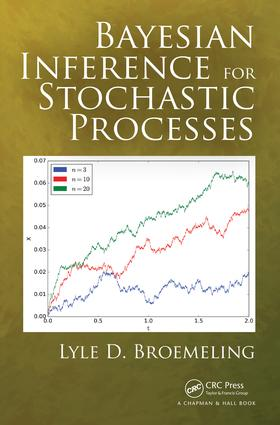# Bayesian Inference for Stochastic Processes

## 1st Edition

Chapman and Hall/CRC

432 pages | 30 B/W Illus.

##### Purchasing Options:\$ = USD
Hardback: 9781138196131
pub: 2017-12-15
SAVE ~\$31.00
\$155.00
\$124.00
x
eBook (VitalSource) : 9781315303598
pub: 2017-12-12
from \$28.98

FREE Standard Shipping!

### Description

This is the first book designed to introduce Bayesian inference procedures for stochastic processes. There are clear advantages to the Bayesian approach (including the optimal use of prior information). Initially, the book begins with a brief review of Bayesian inference and uses many examples relevant to the analysis of stochastic processes, including the four major types, namely those with discrete time and discrete state space and continuous time and continuous state space. The elements necessary to understanding stochastic processes are then introduced, followed by chapters devoted to the Bayesian analysis of such processes. It is important that a chapter devoted to the fundamental concepts in stochastic processes is included. Bayesian inference (estimation, testing hypotheses, and prediction) for discrete time Markov chains, for Markov jump processes, for normal processes (e.g. Brownian motion and the Ornstein–Uhlenbeck process), for traditional time series, and, lastly, for point and spatial processes are described in detail. Heavy emphasis is placed on many examples taken from biology and other scientific disciplines. In order analyses of stochastic processes, it will use R and WinBUGS.

Features:

• Uses the Bayesian approach to make statistical Inferences about stochastic processes
• The R package is used to simulate realizations from different types of processes
• Based on realizations from stochastic processes, the WinBUGS package will provide the Bayesian analysis (estimation, testing hypotheses, and prediction) for the unknown parameters of stochastic processes
• To illustrate the Bayesian inference, many examples taken from biology, economics, and astronomy will reinforce the basic concepts of the subject
• A practical approach is implemented by considering realistic examples of interest to the scientific community
• WinBUGS and R code are provided in the text, allowing the reader to easily verify the results of the inferential procedures found in the many examples of the book

Readers with a good background in two areas, probability theory and statistical inference, should be able to master the essential ideas of this book.

### Reviews

"Readers with a good background in the two areas, probability theory and statistical inference, should be able to master the essential ideas of this book."~ Ludwig Paditz, Dresden

". . .All three important types of Bayesian inferences such are estimation, hypothesis testing and forecasting are considered and many examples are worked through using R and WinBUGS codes. . . It will prove useful for students and scientists who want to learn about Bayesian analysis in stochastic processes." ~Miroslav M. Ristic, Stat Papers

### Table of Contents

1. Introduction to Bayesian Inference for Stochastic Processes

2. Bayesian Analysis

3. Introduction to Stochastic Processes

4. Bayesian Inference for Discrete Markov Chains

5. Examples of Markov Chains in Biology

6. Inferences for Markov Chains in Continuous Time

7. Bayesian Inference: Examples of Continuous-Time Markov Chains

8. Bayesian Inferences for Normal Processes

9. Queues and Time Series

### About the Author

Lyle D. Broemeling, Ph.D., is Director of Broemeling and Associates Inc., and is a consulting biostatistician. He has been involved with academic health science centers for about 20 years and has taught and been a consultant at the University of Texas Medical Branch in Galveston, The University of Texas MD Anderson Cancer Center and the University of Texas School of Public Health. His main interest is in developing Bayesian methods for use in medical and biological problems and in authoring textbooks in statistics. His previous books are Bayesian Biostatistics and Diagnostic Medicine, and Bayesian Methods for Agreement

### Subject Categories

##### BISAC Subject Codes/Headings:
MAT029000
MATHEMATICS / Probability & Statistics / General
MAT029010
MATHEMATICS / Probability & Statistics / Bayesian Analysis